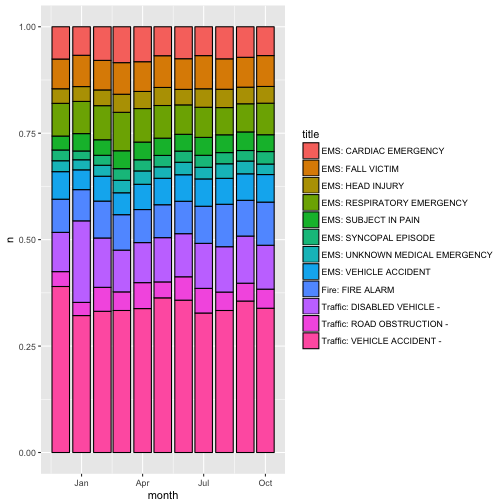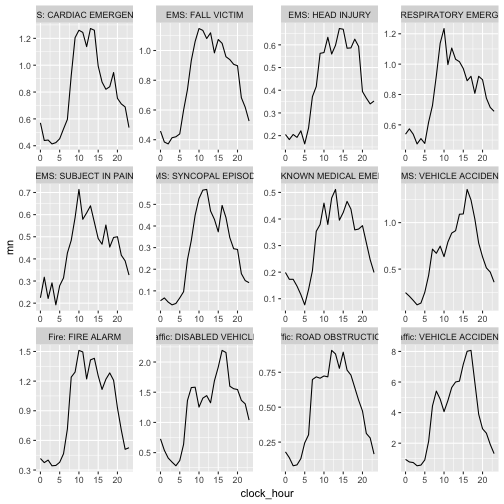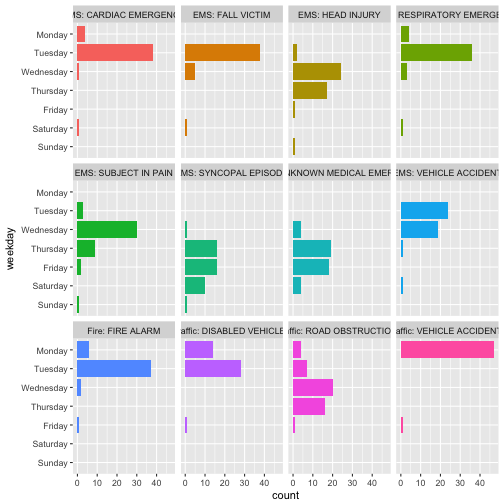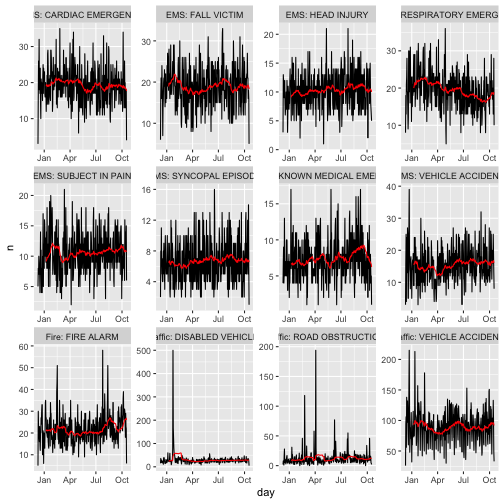# Preparing Datetime Data for Analysis with padr and dplyrTwo months ago padr was introduced, followed by an improved version that allowed for applying pad on group level. See the introduction blogs or the vignette("padr") for more package information. In this blog I give four more elaborate examples on how to go from raw data to insight with padr, dplyr and ggplot2. They might serve as recipes for time series problems you want to solve. The dataset emergency is available in padr, and contains about a year of emergency data in Montgomery County, PA. For this blog I only use the twelve most prevalent emergencies.

library(tidyverse)
top12 <- emergency %>% count(title) %>% arrange(desc(n)) %>% slice(1:12) %>%
select(title) %>% inner_join(emergency)


# 1) A proportion plot over the months

Lets start with a fairly simple problem, we are calculating the relative distribution of events within each month. We normalize the counts within each month, and then plot through time. geom_bar does most of the heavy lifiting here, we just need the months counts for each event. (I checked, all events have calls in each month, there is no need for padding.)

top12 %>% thicken('month', col = 'month') %>%
count(title, month) %>%
ggplot(aes(month, n)) +
geom_bar(aes(fill = title),
col = "black",
stat = 'identity',
position = "fill")## 2) Get a distribution, per event, per time unit

For each clock hour, for each event, we want to compute the average of the number of occurences. The first step is to make the hourly distribution out of the raw data. For every hour we need to count the number of calls per event. This would give no records if there were no calls within a givin hour. If we did not account for this, the calculated averages would be too high. (Recently called “the zero-bug” in this blog post). Therefore we use pad and fill_by_value to insert records and give them the value 0.

hourly_distribution <- top12 %>% thicken('hour') %>%
count(title, time_stamp_hour) %>%


Next step is extracting the clock hour from each timestamp, and calculate the mean per event, per clock hour.

hourly_distribution %>%
mutate(clock_hour = lubridate::hour(time_stamp_hour) ) %>%
group_by(clock_hour, title) %>%
summarise(mn = mean(n)) %>%
ggplot(aes(clock_hour, mn)) +
geom_line() +
facet_wrap(~title, scales = 'free')## 3) Get the 30th observation per time unit

For this dataset it might not be terribly interesting, but there are many situations were it is very useful to extract the first, last, or nth observation per time unit. Lets find the 30th observation per week, where weeks are starting on Mondays. We need an offset in thicken here, to make the weeks start on Mondays.

first_day <- top12\$time_stamp %>% as.Date %>% min
first_day %>% weekdays

##  "Thursday"

top12 %>% thicken('week', start_val = first_day - 3) %>%
group_by(title, time_stamp_week) %>%
filter(min_rank(time_stamp) == 30) %>%
mutate(weekday = weekdays(time_stamp) %>%
factor(levels = (c('Monday', 'Tuesday', 'Wednesday', 'Thursday', 'Friday',
'Saturday', 'Sunday') %>% rev) )) %>%
ggplot(aes(weekday)) +
geom_bar(aes(fill = title)) +
coord_flip() +
guides(fill = FALSE) +
facet_wrap(~title)So here we have a distribution on which day the 30th call came in, for each of the twelve events.

## 4) Make a moving average

Moving averages are very helpful for smoothing time series. It is often a better indication of the underlying trend than the raw data. I recently learned about the RcppRoll package, when I was browsing through R for Data Science. This is a nice package by Kevin Ushey, that makes it terribly easy to calculate rolling stats on a vector. Here we want the moving average of the daily count of each of the events.

top12 %>% thicken('day', col = 'day') %>%
count(day, title) %>%
pad(group = 'title') %>% fill_by_value(n) %>%
group_by(title) %>%
mutate(moving_average = RcppRoll::roll_meanr(x = n, n = 30, fill = NA)) %>%
ggplot(aes(day, n)) +
geom_line() +
geom_line(aes(y = moving_average), col = 'red') +
facet_wrap(~title, scales = 'free')There we have some recipes for preparing data containing timestamp for analysis. Do you have a time series-related challenge not adressed here? I would love to hear from you, and try to figure out an effective way for solving it! Just send an email or post a comment.